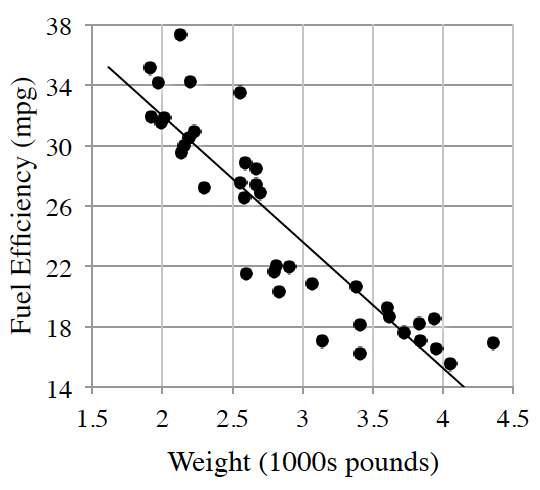### Home > GB8I > Chapter 2 Unit 2 > Lesson INT1: 2.2.3 > Problem2-73

2-73.

A consumer magazine collected the following data for the fuel efficiency of cars (miles per gallon) compared to weight (thousands of pounds).

$e=49−8.4w$
$w$
is the weight ($1000\text{s}$ of pounds)
and $e$ is the fuel efficiency (mpg).

1. What does the $−8.4$ in the equation represent? What are the units on this number?

This is a linear equation.

$−8.4$ is the slope of the line of best fit.
Its units are mpg/$1000$ pounds.

2. What does the 49 in the equation represent? What are its units?

Consider Hint (a).

3. Describe the association between fuel efficiency and weight.

Moderately strong negative linear association with no apparent outliers.

4. Cheetah Motors has come out with a super lightweight sports utility vehicle (SUV) that weighs only $2800$pounds. What does the model predict the fuel efficiency will be?

Use the graph to come up with a prediction of the fuel efficiency of the new SUV.